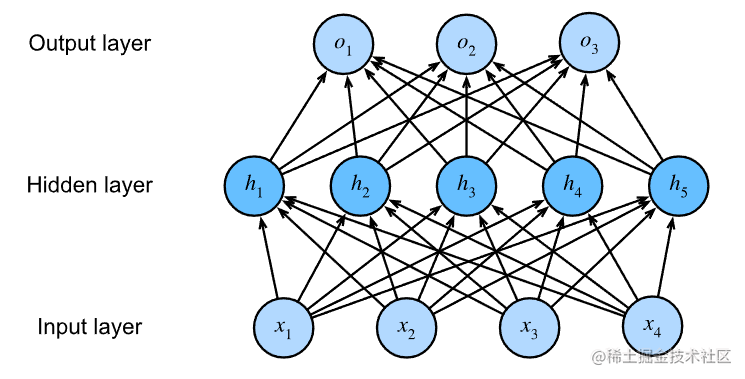# 动手学深度学习4.3 多层感知机的简洁实现``````import torch
from torch import nn
from d2l import torch as d2l

``````net = nn.Sequential(nn.Flatten(),
nn.Linear(784, 256),
nn.ReLU(),
nn.Linear(256, 10))

def init_weights(m):
if type(m) == nn.Linear:
nn.init.normal_(m.weight, std=0.01)

net.apply(init_weights);

• `net = `
• 设定模型，先对输入进行展开，将其变换为向量
• 输入层到隐藏层是784→256
• 设定ReLU层激活函数
• 设定隐藏层到输出层
• `init_weights`给每一层初始化权重
• `init_weights`应用到net上。
``````batch_size, lr, num_epochs = 256, 0.1, 10
loss = nn.CrossEntropyLoss()
trainer = torch.optim.SGD(net.parameters(), lr=lr)

• 这里batch_size是设定mini-batch的批量大小为256，lr设定learning-rate为0.1，num_epochs设定迭代次数为10
• 训练过程也是直接使用框架自带的SGD
``````train_iter, test_iter = d2l.load_data_fashion_mnist(batch_size)
d2l.train_ch3(net, train_iter, test_iter, loss, num_epochs, trainer)